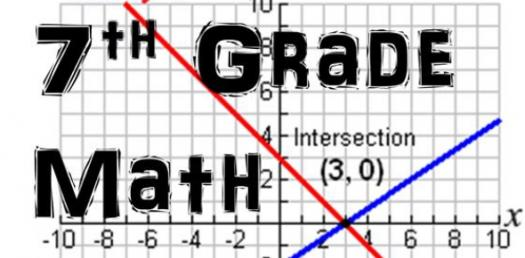# 7th Grade Math Vocabulary Test (Angles And Lines)

17 Questions | Total Attempts: 628SettingsCreate your own QuizMost people do not have a strong love for all things mathematics, but that love can grow slowly by slowly. Over the past week in seventh grade, we got to cover angles and graphs and the workings associated with it. Were you paying attention and think you understood what we covered? Test your math vocabulary on the topic by taking this test. All the best!

• 1.
Congruent
• A.

Same size, shape, and position

• B.

Same size and shape

• C.

Same size only

• D.

Same shape only

• 2.
Point
• A.

A straight path that extends infinitely in both directions

• B.

A completely flat plane that extends in all directions

• C.

Part of a line that extends infinitely in one direction

• D.

A dot that represents a location

• 3.
Plane
• A.

A completely flat surface that extends in all directions

• B.

A 3-dimenstional base that extends in all directions

• C.

A dot that represents a location

• D.

A line that represents a location

• 4.
Line
• A.

A dot that represents a location

• B.

A straight path that is infinite in both directions

• C.

Connects 2 points

• D.

A straight path that extends infinitely in one direction

• 5.
Line Segment
• A.

A straight path that extends infinitely in both directions

• B.

A completely flat plane that extends in all directions

• C.

Part of a line that extends infinitely in one direction

• D.

Connects 2 endpoints

• 6.
Ray
• A.

A straight path that is infinite in both directions

• B.

A line segment that connects 2 points

• C.

Part of a line that extends infinitely in one direction

• D.

A crooked path that splits in the middle

• 7.
Now we're continuing on to the angle types! :D
• A.

Cool. :) When do we start?

• B.

Okay. Why is this a question?

• C.

I really want to get this overwith...

• D.

Whatever...

• 8.
Vertical angle
• A.

Angles that are congruent lines that cross

• B.

Angles that cross, but aren't perpindicular

• C.

Congruent angles that are on the same side of transversals

• D.

Angles that don't touch, and are parallel

• 9.
• A.

Angles that are congruent lines that cross

• B.

Angles that cross, but aren't perpindicular

• C.

Congruent angles that are on the same side of transversals

• D.

Angles that don't touch, and are parallel

• 10.
Complementary Angles
• A.

Angles that total 45 degrees

• B.

Angles that total 80 degrees

• C.

Angles that total 90 degrees

• D.

Angles that total 180 degrees

• E.

Angles that total 360 degrees

• 11.
Supplementary Angles
• A.

Angles that total 45 degrees

• B.

Angles that total 80 degrees

• C.

Angles that total 90 degrees

• D.

Angles that total 180 degrees

• E.

Angles that total 360 degrees

• 12.
Vertical angle
• A.

Angles that are congruent lines that cross

• B.

Angles that cross, but aren't perpindicular

• C.

Congruent angles that are on the same side of transversals

• D.

Angles that don't touch, and are parallel

• 13.
Guess what???
• A.

We're moving on to angle types? 8D

• B.

Something fun...?

• C.

How am I supposed to know? ^^"

• D.

Why bother...

• 14.
Did you know that we're doing line types next?? (:
• A.

What?? No way! :O I'm psyched!

• B.

Yup! I read the description.

• C.

Nope. No, I didn't.. -_-

• 15.
I guess that's all! Did you like the quiz?
• A.

Yes! It was the most awesome quiz..EVER!

• B.

Not really, but it helped me to study for my test.

• C.

Sure, I guess?

• D.

No. It was boring and stupid...kind of like math class all over again!

Related TopicsBack to top
×

Wait!
Here's an interesting quiz for you.